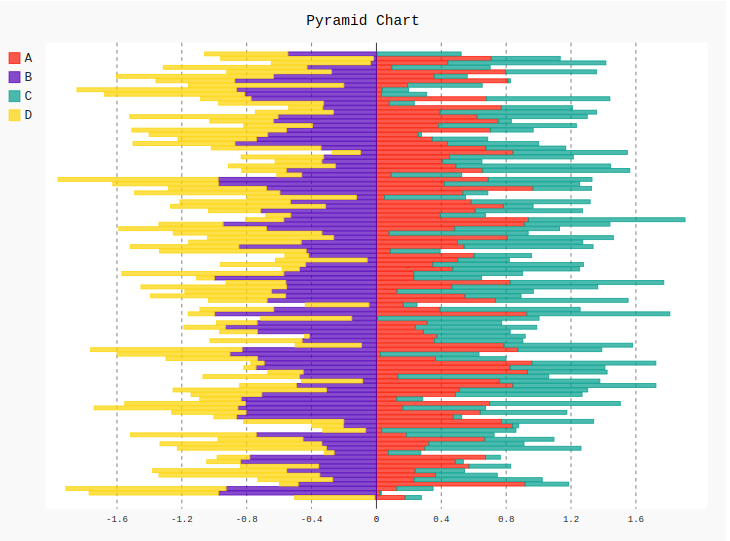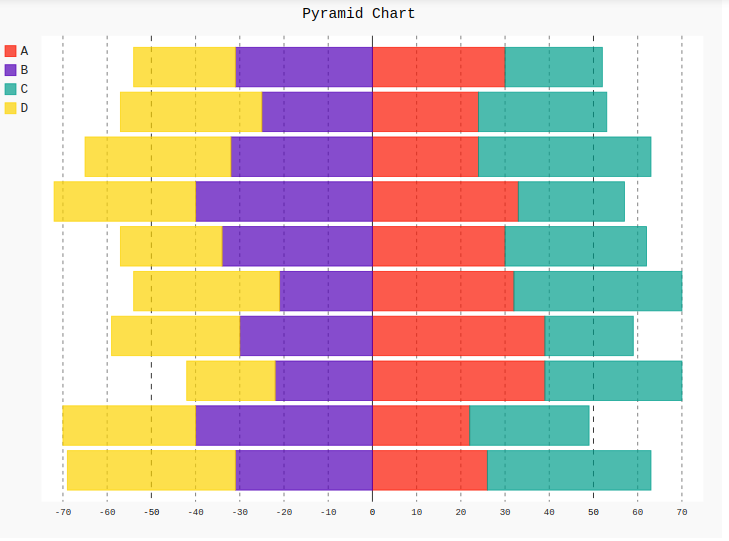# Pyramid chart in pygal

Pygal is a Python module that is mainly used to build SVG (Scalar Vector Graphics) graphs and charts. SVG is a vector-based graphics in the XML format that can be edited in any editor. Pygal can create graphs with minimal lines of code that can be easy to understand and write.

## Pyramid Chart

The pyramid graph is a chart whose shape is a triangle or pyramid. These charts are beneficial in use when the data is organized in some kind of apparatus way. The increase or decrease of the pyramid indicates some kind of progressive order like more important to least important. It can be created using the Pyramid method.

Syntax:

`pyramid_chart = pygal.Pyramid()`

Example 1:

 `import` `pygal ` `import` `random ` ` `  `# Random Data ` `data ``=` `[numpy.random.rand(``100``), numpy.random.rand(``100``), ` `        ``numpy.random.rand(``100``), numpy.random.rand(``100``)] ` ` `  `types ``=` `[``'A'``, ``'B'``, ` `         ``'C'``, ``'D'``, ] ` ` `  `pyramid_chart ``=` `pygal.Pyramid() ` ` `  `# Naming the title ` `pyramid_chart.title ``=` `'Pyramid Chart'` ` `  `for` `type``, dat ``in` `zip``(types, data): ` `    ``pyramid_chart.add(``type``, dat) ` ` `  `pyramid_chart `

Output:Example 2:

 `import` `pygal ` `import` `random ` ` `  ` `  `# Random Data ` `data ``=` `[[``26``, ``22``, ``39``, ``39``, ``32``, ``30``, ``33``, ``24``, ``24``, ``30``],  ` `        ``[``31``, ``40``, ``22``, ``30``, ``21``, ``34``, ``40``, ``32``, ``25``, ``31``],  ` `        ``[``37``, ``27``, ``31``, ``20``, ``38``, ``32``, ``24``, ``39``, ``29``, ``22``],  ` `        ``[``38``, ``30``, ``20``, ``29``, ``33``, ``23``, ``32``, ``33``, ``32``, ``23``]] ` ` `  `types ``=` `[``'A'``, ``'B'``, ` `         ``'C'``, ``'D'``, ] ` ` `  `pyramid_chart ``=` `pygal.Pyramid() ` ` `  `# Naming the title ` `pyramid_chart.title ``=` `'Pyramid Chart'` ` `  `for` `type``, dat ``in` `zip``(types, data): ` `    ``pyramid_chart.add(``type``, dat) ` ` `  `pyramid_chart `

Output:My Personal Notes arrow_drop_upIf you like GeeksforGeeks and would like to contribute, you can also write an article using contribute.geeksforgeeks.org or mail your article to contribute@geeksforgeeks.org. See your article appearing on the GeeksforGeeks main page and help other Geeks.

Please Improve this article if you find anything incorrect by clicking on the "Improve Article" button below.

Article Tags :

Be the First to upvote.

Please write to us at contribute@geeksforgeeks.org to report any issue with the above content.